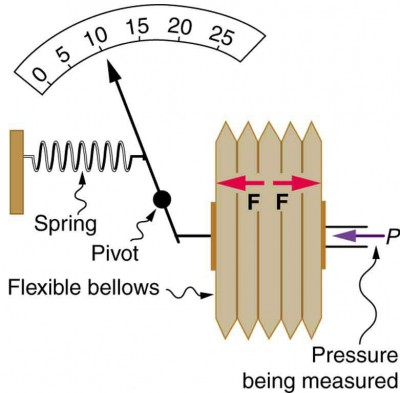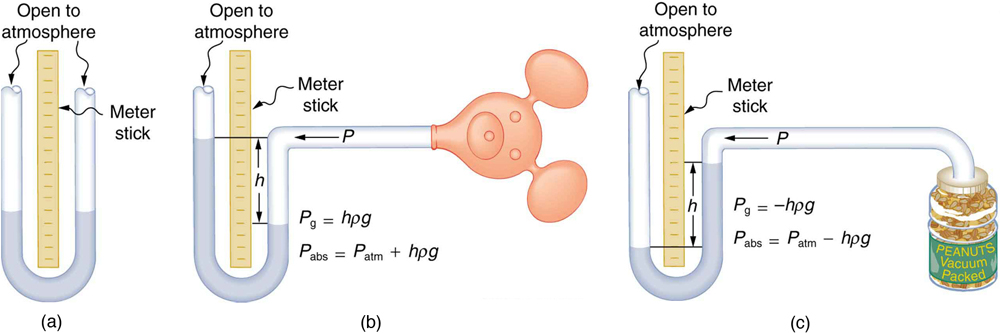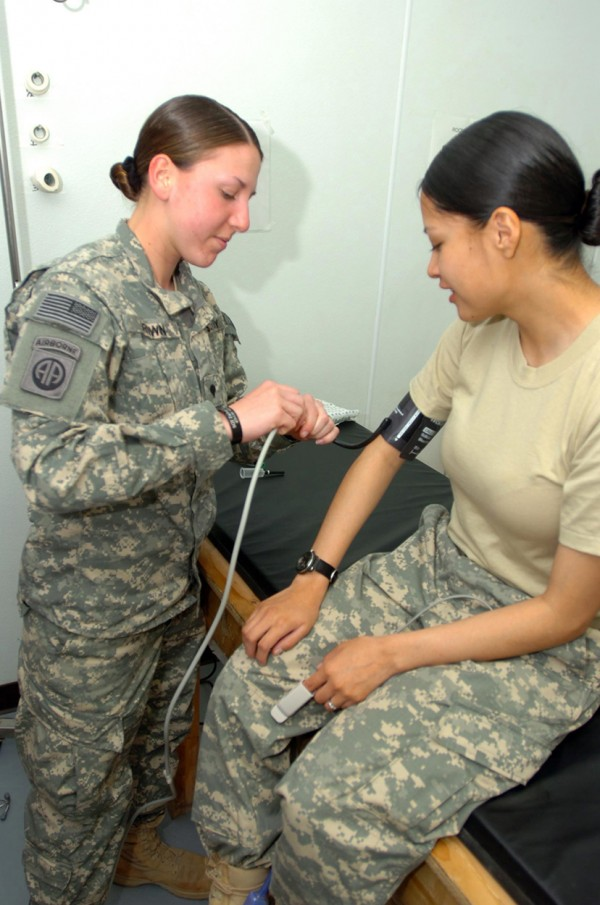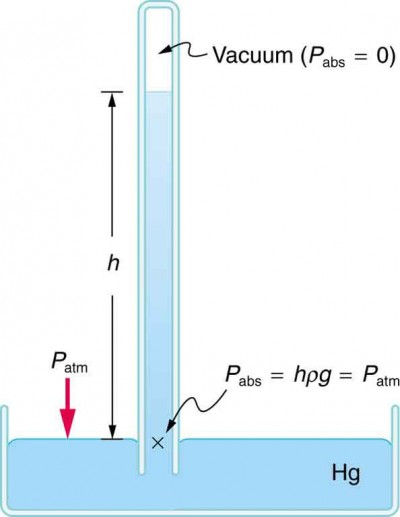Gauge Pressure, Absolute Pressure, and Pressure Measurement

Learning Objectives

By the end of this section, you will be able to:

• Define gauge pressure and absolute pressure.
• Understand the working of aneroid and open-tube barometers.

If you limp into a gas station with a nearly flat tire, you will notice the tire gauge on the airline reads nearly zero when you begin to fill it. In fact, if there were a gaping hole in your tire, the gauge would read zero, even though atmospheric pressure exists in the tire. Why does the gauge read zero? There is no mystery here. Tire gauges are simply designed to read zero at atmospheric pressure and positive when pressure is greater than atmospheric.

Similarly, atmospheric pressure adds to blood pressure in every part of the circulatory system. (As noted in Pascal’s Principle, the total pressure in a fluid is the sum of the pressures from different sources—here, the heart and the atmosphere.) But atmospheric pressure has no net effect on blood flow since it adds to the pressure coming out of the heart and going back into it, too. What is important is how much greater blood pressure is than atmospheric pressure. Blood pressure measurements, like tire pressures, are thus made relative to atmospheric pressure.

In brief, it is very common for pressure gauges to ignore atmospheric pressure—that is, to read zero at atmospheric pressure. We therefore define gauge pressure to be the pressure relative to atmospheric pressure. Gauge pressure is positive for pressures above atmospheric pressure, and negative for pressures below it.

Gauge Pressure

Gauge pressure is the pressure relative to atmospheric pressure. Gauge pressure is positive for pressures above atmospheric pressure, and negative for pressures below it.

In fact, atmospheric pressure does add to the pressure in any fluid not enclosed in a rigid container. This happens because of Pascal’s principle. The total pressure, or absolute pressure, is thus the sum of gauge pressure and atmospheric pressure: Pabs PPatm where Pabs is absolute pressure, Pg is gauge pressure, and Patm is atmospheric pressure. For example, if your tire gauge reads 34 psi (pounds per square inch), then the absolute pressure is 34 psi plus 14.7 psi (Patm in psi), or 48.7 psi (equivalent to 336 kPa).

Absolute Pressure

Absolute pressure is the sum of gauge pressure and atmospheric pressure.

For reasons we will explore later, in most cases the absolute pressure in fluids cannot be negative. Fluids push rather than pull, so the smallest absolute pressure is zero. (A negative absolute pressure is a pull.) Thus the smallest possible gauge pressure is P= −Patm (this makes Pabs zero). There is no theoretical limit to how large a gauge pressure can be.

There are a host of devices for measuring pressure, ranging from tire gauges to blood pressure cuffs. Pascal’s principle is of major importance in these devices. The undiminished transmission of pressure through a fluid allows precise remote sensing of pressures. Remote sensing is often more convenient than putting a measuring device into a system, such as a person’s artery. Figure 1 shows one of the many types of mechanical pressure gauges in use today. In all mechanical pressure gauges, pressure results in a force that is converted (or transduced) into some type of readout.Figure 1. This aneroid gauge utilizes flexible bellows connected to a mechanical indicator to measure pressure.

An entire class of gauges uses the property that pressure due to the weight of a fluid is given by hρg. Consider the U-shaped tube shown in Figure 2, for example. This simple tube is called a manometer. In Figure 2(a), both sides of the tube are open to the atmosphere. Atmospheric pressure therefore pushes down on each side equally so its effect cancels. If the fluid is deeper on one side, there is a greater pressure on the deeper side, and the fluid flows away from that side until the depths are equal.

Let us examine how a manometer is used to measure pressure. Suppose one side of the U-tube is connected to some source of pressure Pabs such as the toy balloon in Figure 2(b) or the vacuum-packed peanut jar shown in Figure 2(c). Pressure is transmitted undiminished to the manometer, and the fluid levels are no longer equal. In Figure 2(b), Pabs is greater than atmospheric pressure, whereas in Figure 2(c), Pabs is less than atmospheric pressure. In both cases, Pabs differs from atmospheric pressure by an amount hρg, where ρ is the density of the fluid in the manometer. In Figure 2(b), Pabs can support a column of fluid of height h, and so it must exert a pressure hρg greater than atmospheric pressure (the gauge pressure Pg is positive). In Figure 2(c), atmospheric pressure can support a column of fluid of height h, and so Pabs is less than atmospheric pressure by an amount hρg (the gauge pressure Pg is negative). A manometer with one side open to the atmosphere is an ideal device for measuring gauge pressures. The gauge pressure is Phρg and is found by measuring h.Figure 2. An open-tube manometer has one side open to the atmosphere. (a) Fluid depth must be the same on both sides, or the pressure each side exerts at the bottom will be unequal and there will be flow from the deeper side. (b) A positive gauge pressure P= hρg transmitted to one side of the manometer can support a column of fluid of height h. (c) Similarly, atmospheric pressure is greater than a negative gauge pressure Pg by an amount hρg. The jar’s rigidity prevents atmospheric pressure from being transmitted to the peanuts.

Mercury manometers are often used to measure arterial blood pressure. An inflatable cuff is placed on the upper arm as shown in Figure 3. By squeezing the bulb, the person making the measurement exerts pressure, which is transmitted undiminished to both the main artery in the arm and the manometer. When this applied pressure exceeds blood pressure, blood flow below the cuff is cut off. The person making the measurement then slowly lowers the applied pressure and listens for blood flow to resume. Blood pressure pulsates because of the pumping action of the heart, reaching a maximum, called systolic pressure, and a minimum, called diastolic pressure, with each heartbeat. Systolic pressure is measured by noting the value of h when blood flow first begins as cuff pressure is lowered. Diastolic pressure is measured by noting h when blood flows without interruption. The typical blood pressure of a young adult raises the mercury to a height of 120 mm at systolic and 80 mm at diastolic. This is commonly quoted as 120 over 80, or 120/80. The first pressure is representative of the maximum output of the heart; the second is due to the elasticity of the arteries in maintaining the pressure between beats. The density of the mercury fluid in the manometer is 13.6 times greater than water, so the height of the fluid will be 1/13.6 of that in a water manometer. This reduced height can make measurements difficult, so mercury manometers are used to measure larger pressures, such as blood pressure. The density of mercury is such that 1.0 mm Hg = 133 Pa.

Systolic Pressure

Systolic pressure is the maximum blood pressure.

Diastolic Pressure

Diastolic pressure is the minimum blood pressure.Figure 3. In routine blood pressure measurements, an inflatable cuff is placed on the upper arm at the same level as the heart. Blood flow is detected just below the cuff, and corresponding pressures are transmitted to a mercury-filled manometer. (credit: U.S. Army photo by Spc. Micah E. Clare4TH BCT)

Example 1. Calculating Height of IV Bag: Blood Pressure and Intravenous Infusions

Intravenous infusions are usually made with the help of the gravitational force. Assuming that the density of the fluid being administered is 1.00 g/ml, at what height should the IV bag be placed above the entry point so that the fluid just enters the vein if the blood pressure in the vein is 18 mm Hg above atmospheric pressure? Assume that the IV bag is collapsible.

Strategy for (a)

For the fluid to just enter the vein, its pressure at entry must exceed the blood pressure in the vein (18 mm Hg above atmospheric pressure). We therefore need to find the height of fluid that corresponds to this gauge pressure.

Solution

We first need to convert the pressure into SI units. Since 1.0 mm Hg = 133 Pa,

$P=\text{18 mm Hg}\times \frac{\text{133 Pa}}{1.0 \text{ mm Hg}}=\text{2400 Pa}\\$

Rearranging Phρg for h gives $h=\frac{{P}_{\text{g}}}{\mathrm{\rho g}}\\$. Substituting known values into this equation gives

$\begin{array}{lll}h& =& \frac{\text{2400 N}{\text{/m}}^{2}}{\left(1\text{.}0\times {\text{10}}^{3}{\text{kg/m}}^{3}\right)\left(9\text{.}\text{80}{\text{m/s}}^{2}\right)}\\ & =& \text{0.24 m.}\end{array}\\$

Discussion

The IV bag must be placed at 0.24 m above the entry point into the arm for the fluid to just enter the arm. Generally, IV bags are placed higher than this. You may have noticed that the bags used for blood collection are placed below the donor to allow blood to flow easily from the arm to the bag, which is the opposite direction of flow than required in the example presented here.

A barometer is a device that measures atmospheric pressure. A mercury barometer is shown in Figure 4. This device measures atmospheric pressure, rather than gauge pressure, because there is a nearly pure vacuum above the mercury in the tube. The height of the mercury is such that hρg Patm. When atmospheric pressure varies, the mercury rises or falls, giving important clues to weather forecasters. The barometer can also be used as an altimeter, since average atmospheric pressure varies with altitude. Mercury barometers and manometers are so common that units of mm Hg are often quoted for atmospheric pressure and blood pressures. Table 1 gives conversion factors for some of the more commonly used units of pressure.Figure 4. A mercury barometer measures atmospheric pressure. The pressure due to the mercury’s weight, hρg, equals atmospheric pressure. The atmosphere is able to force mercury in the tube to a height h because the pressure above the mercury is zero.

Table 1. Conversion Factors for Various Pressure Units
Conversion to N/m2 (Pa) Conversion from atm
1.0 atm = 1.013 × 105 N/m2 1.0 atm = 1.013 × 105 N/m2
1.0 dyne/cm2 = 0.10 N/m2 1.0 atm = 1.013 × 106 dyne/cm2
1.0 kg/cm2 = 9.8 × 104 N/m2 1.0 atm = 1.013 kg/cm2
1.0 lb/in.2 = 6.90 × 103 N/m2 1.0 atm = 14.7 lb/in.2
1.0 mm Hg = 133 N/m2 1.0 atm = 760 mm Hg
1.0 cm Hg = 1.33 × 103 N/m2 1.0 atm = 76.0 cm Hg
1.0 cm water = 98.1 N/m2 1.0 atm = 1.03 × 103 cm water
1.0 bar = 1.000 × 105 N/m2 1.0 atm = 1.013 bar
1.0 millibar = 1.000 × 102 N/m2 1.0 atm = 1013 millibar

Section Summary

• Gauge pressure is the pressure relative to atmospheric pressure.
• Absolute pressure is the sum of gauge pressure and atmospheric pressure.
• Aneroid gauge measures pressure using a bellows-and-spring arrangement connected to the pointer of a calibrated scale.
• Open-tube manometers have U-shaped tubes and one end is always open. It is used to measure pressure.
• A mercury barometer is a device that measures atmospheric pressure.

Conceptual Questions

1. Explain why the fluid reaches equal levels on either side of a manometer if both sides are open to the atmosphere, even if the tubes are of different diameters.

2. Figure 3 shows how a common measurement of arterial blood pressure is made. Is there any effect on the measured pressure if the manometer is lowered? What is the effect of raising the arm above the shoulder? What is the effect of placing the cuff on the upper leg with the person standing? Explain your answers in terms of pressure created by the weight of a fluid.

3. Considering the magnitude of typical arterial blood pressures, why are mercury rather than water manometers used for these measurements?

Problems & Exercises

1. Find the gauge and absolute pressures in the balloon and peanut jar shown in Figure 2, assuming the manometer connected to the balloon uses water whereas the manometer connected to the jar contains mercury. Express in units of centimeters of water for the balloon and millimeters of mercury for the jar, taking h = 0.0500 m for each.Figure 2. An open-tube manometer has one side open to the atmosphere. (a) Fluid depth must be the same on both sides, or the pressure each side exerts at the bottom will be unequal and there will be flow from the deeper side. (b) A positive gauge pressure P= hρg transmitted to one side of the manometer can support a column of fluid of height h. (c) Similarly, atmospheric pressure is greater than a negative gauge pressure Pg by an amount hρg. The jar’s rigidity prevents atmospheric pressure from being transmitted to the peanuts.

2. (a) Convert normal blood pressure readings of 120 over 80 mm Hg to newtons per meter squared using the relationship for pressure due to the weight of a fluid $\left(P={h\rho g}\right)\\$ rather than a conversion factor. (b) Discuss why blood pressures for an infant could be smaller than those for an adult. Specifically, consider the smaller height to which blood must be pumped.

3. How tall must a water-filled manometer be to measure blood pressures as high as 300 mm Hg?

4. Pressure cookers have been around for more than 300 years, although their use has strongly declined in recent years (early models had a nasty habit of exploding). How much force must the latches holding the lid onto a pressure cooker be able to withstand if the circular lid is 25.0 cm in diameter and the gauge pressure inside is 300 atm? Neglect the weight of the lid.

5. Suppose you measure a standing person’s blood pressure by placing the cuff on his leg 0.500 m below the heart. Calculate the pressure you would observe (in units of mm Hg) if the pressure at the heart were 120 over 80 mm Hg. Assume that there is no loss of pressure due to resistance in the circulatory system (a reasonable assumption, since major arteries are large).

6. A submarine is stranded on the bottom of the ocean with its hatch 25.0 m below the surface. Calculate the force needed to open the hatch from the inside, given it is circular and 0.450 m in diameter. Air pressure inside the submarine is 1.00 atm.

7. Assuming bicycle tires are perfectly flexible and support the weight of bicycle and rider by pressure alone, calculate the total area of the tires in contact with the ground. The bicycle plus rider has a mass of 80.0 kg, and the gauge pressure in the tires is 3.50 × 10Pa.

Glossary

absolute pressure:
the sum of gauge pressure and atmospheric pressure
diastolic pressure:
the minimum blood pressure in the artery
gauge pressure:
the pressure relative to atmospheric pressure
systolic pressure:
the maximum blood pressure in the artery

Selected Solutions to Problems & Exercises

1. Balloon:

Pg = 5.00 cm H2O,

Pabs = 1.035 × 103 cm H2O

Jar:

Pg = -50.0 mm Hg,

Pabs = 710 mm Hg.

3. 4.08 m

5. $\begin{array}{}\Delta P=\text{38.7 mm Hg,}\\ \text{Leg blood pressure}=\frac{\text{159}}{\text{119}}\end{array}\\$

7. 22.4 cm2